# The 18th International Mathematical Olympiad COLIN GOLDSMITHMarlborough College

The team from Great Britain to the olympiad held in Lienz, Austria in July 1976 included four who had competed previously, so it was expected to do well. In the event, we achieved our highest place ever, second to the Soviet Union.

The competition, for teams of up to eight pre-university students, consists of three questions to be tackled in a four-hour session on one day followed by three more questions in a similar session on the following day. Correct answers are not sufficient; close reasoning is required and points are lost for any lapses in the rigour of a proof. No charity is shown, with the result that only one student, a Frenchman, scored full marks, one, a Russian girl, obtained 39 points and 36 obtained a single-figure total out of a maximum of 40 points.

The results showing total scores for each team of eight competitors are tabulated below:

QuestionPrizes
123456Total1st2nd3rd
USSR364646463145250431
Great Britain233552431150214241
USA251832442643188141
Bulgaria2218373665517426
Austria26163428855167125
France213127222242165131
Hungary2715193885316034
East Germany26182827123114223
Poland251510420461386
Sweden190382703612013
Rumania1810132814811813
Czechoslovakia2211133073311613
Yugoslavia2610223442011613
Vietnam2191529102811213
Holland156927516781
Finland931110019521
Greece28141211350
Maximum405664485656320

In addition, Cuba entered three competitors and West Germany made a trial appearance for the first time, with two students.

It is interesting to note how each country fared on each question, the variations reflecting the different mathematics syllabuses in operation. Calculus is not in the school syllabus in many of the Eastern European countries, so no questions requiring calculus are set in the IMO. However, a knowledge of calculus was used to advantage by many competitors in Question 2, and this partly explains the relatively good showing of the British team on this difficult question. On the other hand, one was more likely to find the best solution to Question 4 if no calculus was known. Similarly, some experience of linear algebra proved a real disadvantage in Question 5 which can only be solved successfully by combinatorial methods. A pragmatic approach works well with Question 3, equations and formal argument being used only after considerable numerical investigation; it was here that the British were most effective.

Like other international contests, the IMO aims to foster goodwill and interchange of ideas and information between team members and officials. This year’s olympiad certainly fulfilled these objectives, due in no small part to the beauty of the surroundings in the Tyrol, the imaginative organisation of our Austrian hosts and their exceptional generosity.

All countries face the problem of providing stimulus and suitable tuition for the mathematically gifted, often within a more or less comprehensive system of education and usually without the specialisation we are accustomed to in the sixth form. Some (e.g. the Soviet Union and Yugoslavia) have special mathematical schools. Regional and national competitions go some way to identify and encourage talent, and the chance to represent one’s country on an enjoyable trip abroad is a further carrot. Tuition by correspondence is used in some countries and most hold training sessions for their IMO teams. (The USA team had three weeks preparation together immediately prior to the olympiad.) Here we have in the past provided no special training and the team has met for the first time at the start of the journey to the olympiad. This year for the first time some problems have been sent at intervals by post to those requesting them, solutions being provided with the next problems. Any British school or sixth-former wishing to take advantage of this service should write to Mr R. C. Lyness, Singleton Lodge, Blackpool FY6 8LT, enclosing a stamped self-addressed envelope.

## Appendix 1

The questions in the 1976 olympiad were as follows.

1. (5 points) In a plane convex quadrilateral of area 32 cm2 the sum of the lengths of two opposite sides and one diagonal is equal to 16 cm.

Determine all possible lengths of the other diagonal.

2. (7 points) Let P1(x) = x2 - 2 and Pj(x) = P1(Pj - 1(x)) for j = 2, 3, .... Show that, for any positive integer n, the roots of the equation Pn(x) = x are all real and distinct.

3. (8 points) A rectangular box can be filled completely with unit cubes. If one places as many cubes as possible, each with volume 2, in the box so that their edges are parallel to the edges of the box, one can fill exactly 40 per cent of the box. Determine the interior dimensions of all such boxes. (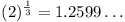.)

4. (6 points) Determine, with proof, the largest number which is the product of positive integers whose sum is 1976.

5. (7 points) Consider the system of p equations in q unknowns, where q = 2p,

a11x1 + ... + a1qxq = 0,
a21x1 + ... + a2qxq = 0,
...
ap1x1 + ... + apqxq = 0,

with every coefficient aij a member of the set { -1, 0, +1 }. Prove that there exists a solution (x1, ..., xq) of the system such that

1. all xj (j = 1, ..., q) are integers;
2. there is at least one value of j for which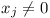;
3.(j = 1, ..., q).
6. (7 points) A sequence {un} is defined by u0 = 2, u1 = 5/2, un + 1 = un(un - 12 - 2) - u1 for n = 1, 2, ....

Prove that, for positive integers n,

[un] = 2p, where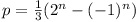,

and [x] denotes the greatest integer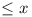.

## Appendix 2

Special prizes may be given by the international jury for solutions of particular merit. One only was awarded this year, and this went to J. R. Rickard (City of London School) for generalising the result of Question 5 in the following way.

If ‘q = 2p’ is replaced by ‘q = kp, k > 1’, and the restriction that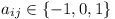is replaced by ‘aij is an integer with,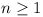’, then there exists a solution satisfying (a), (b) and

(c')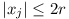, where r is the least integer greater than or equal to ½(nq)1 / (k - 1).

His proof of the original problem, suitably modified for the generalised problem, went as follows.

Consider the ways of assigning -r, -r + 1, ..., -1, 0, 1, ..., r to each of x1, ..., xq. There are (2r + 1)q such ways.

For each of these, L1, ..., Lp, the values of the left-hand sides of the equations are such that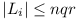. So there are at most (2nqr + 1)p different sets of values for L1, ..., Lp. Now

2r + 1 > (nq)1 / (k - 1),

i.e.

(2r + 1)k - 1 > nq,

and thereforeso that

(2r + 1)q > (2nqr + 1)p.

From the pigeon-hole principle it follows that there exist two distinct sets of values for x1, ..., xq which give the same set of values for L1, ..., Lp. Call these y1, ..., yq and z1, ..., zq. Then Xi = yi - zi is easily seen to give a set of integer values which satisfy the original equations. Moreover,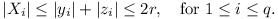Reproduced with permission from Mathematical Spectrum volume 9 (1976–7) pages 37–40